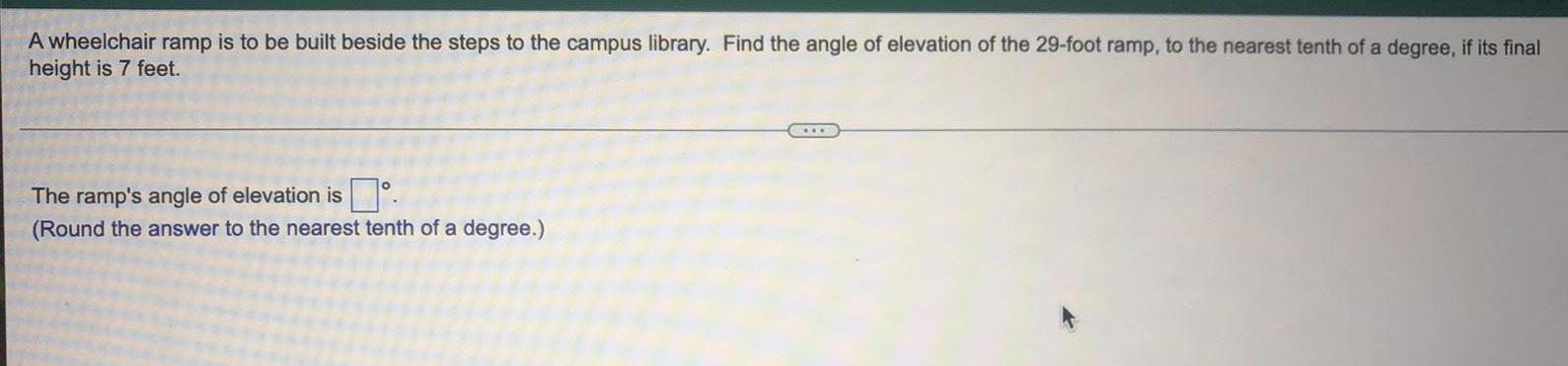Math
Trigonometry
A wheelchair ramp is to be built beside the steps to the campus library. Find the angle of elevation of the 29-foot ramp, to the nearest tenth of a degree, if its final height is 7 feet. The ramp's angle of elevation is (Round the answer to the nearest tenth of a degree.)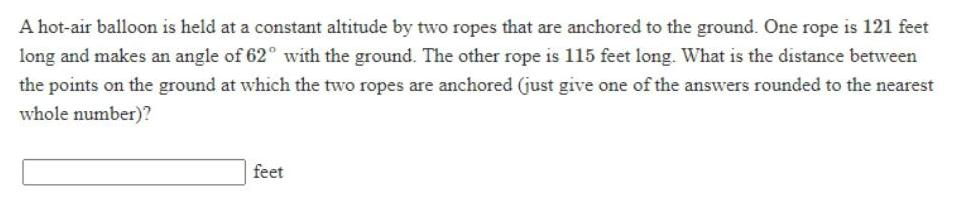Math
Trigonometry
A hot-air balloon is held at a constant altitude by two ropes that are anchored to the ground. One rope is 121 feet long and makes an angle of 62° with the ground. The other rope is 115 feet long. What is the distance between the points on the ground at which the two ropes are anchored (just give one of the answers rounded to the nearest whole number)?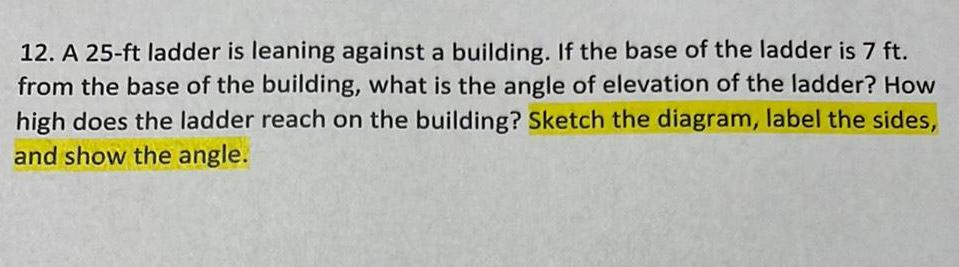Math
Trigonometry
A 25-ft ladder is leaning against a building. If the base of the ladder is 7 ft. from the base of the building, what is the angle of elevation of the ladder? How high does the ladder reach on the building? Sketch the diagram, label the sides, and show the angle.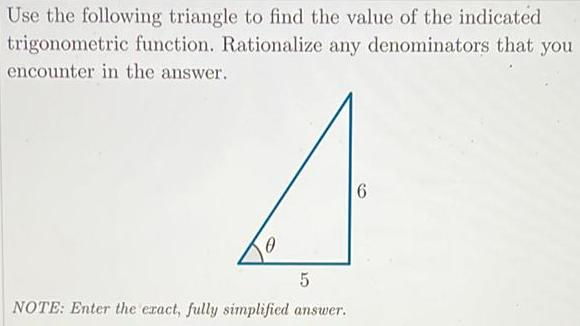Math
Trigonometry
Use the following triangle to find the value of the indicated trigonometric function. Rationalize any denominators that you encounter in the answer.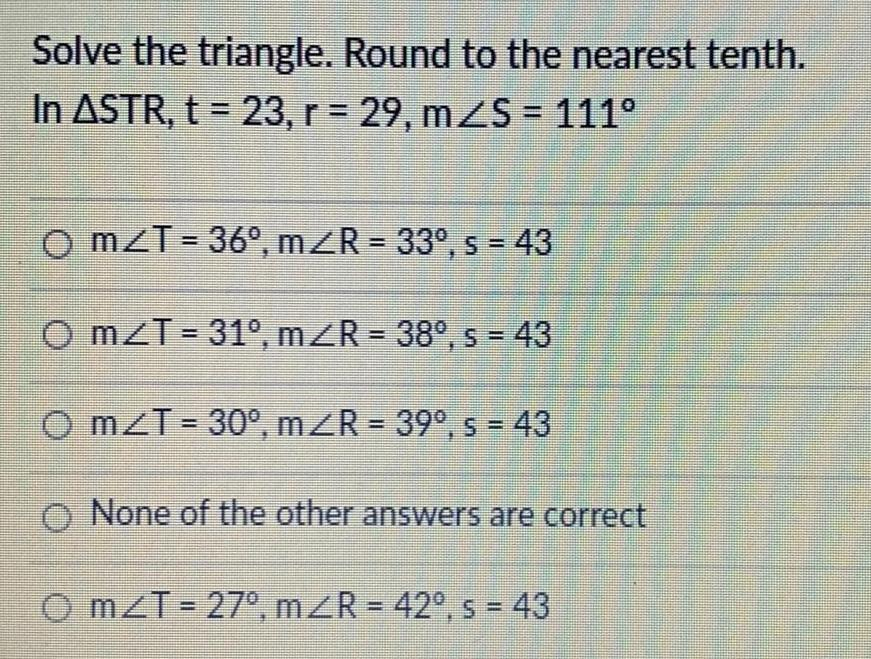Math
Trigonometry
Solve the triangle. Round to the nearest tenth. In ASTR, t = 23, r = 29, mZS = 111° mZT=36⁰, m≤R = 33º, s = 43 mZT=31°, m/R = 38°, s = 43 mZT=30°, m/R = 39°, s = 43 None of the other answers are correct OmZT = 27°, m≤R = 42°, s = 43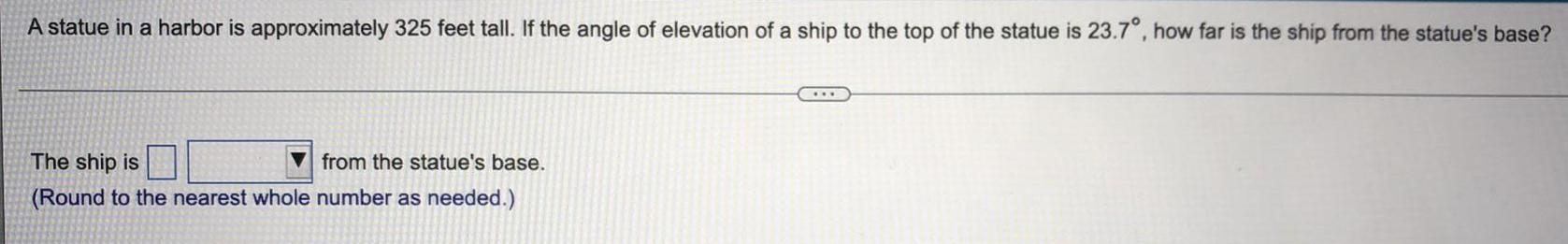Math
Trigonometry
A statue in a harbor is approximately 325 feet tall. If the angle of elevation of a ship to the top of the statue is 23.7°, how far is the ship from the statue's base? The ship is from the statue's base.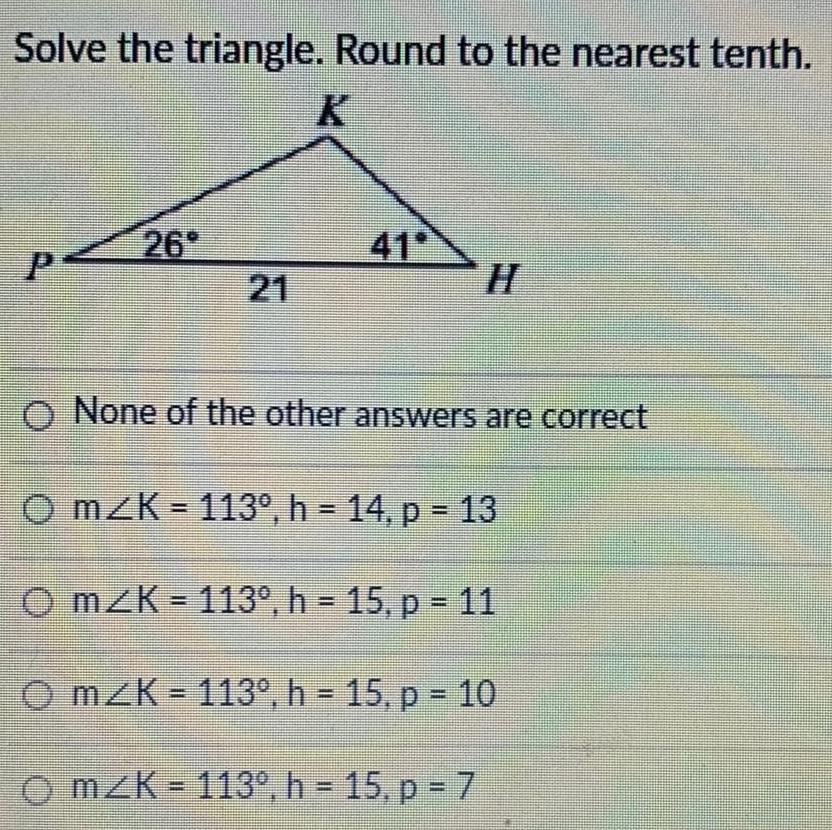Math
Trigonometry
Solve the triangle. Round to the nearest tenth. None of the other answers are correct mZK 113°, h 14, p = 13 mZK 113⁰, h = 15, p = 11 mZK 113°, h = 15, p = 10 mZK-113°, h = 15, p = 7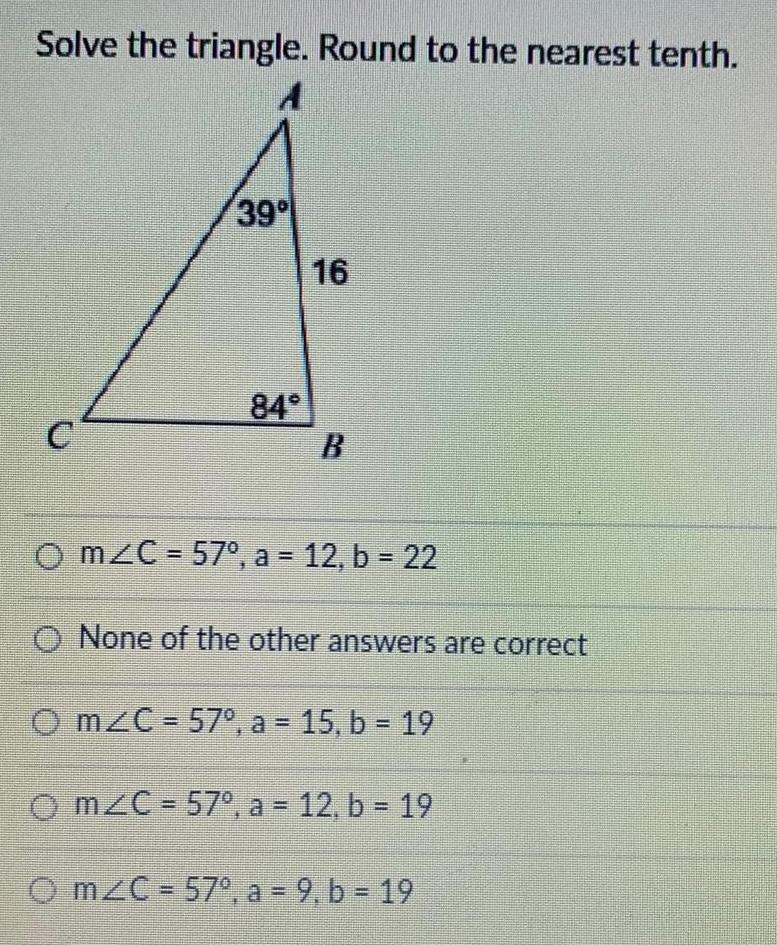Math
Trigonometry
Solve the triangle. Round to the nearest tenth. m/C = 57°, a = 12, b = 22 None of the other answers are correct mZC= 57°, a = 15, b = 19 mZC = 57°, a = 12, b = 19 mZC = 57°, a = 9, b = 19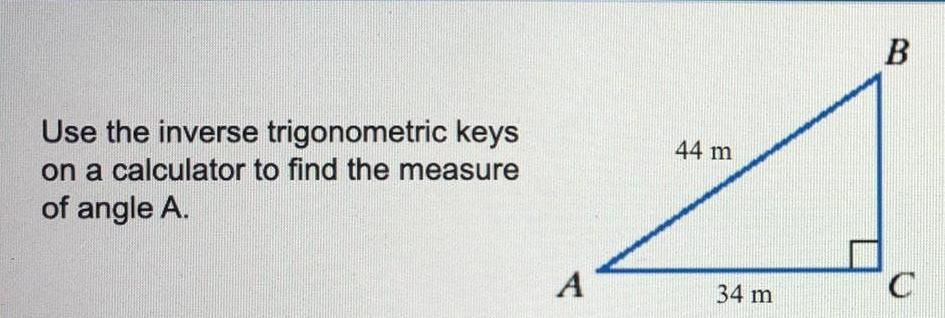Math
Trigonometry
Use the inverse trigonometric keys on a calculator to find the measure of angle A.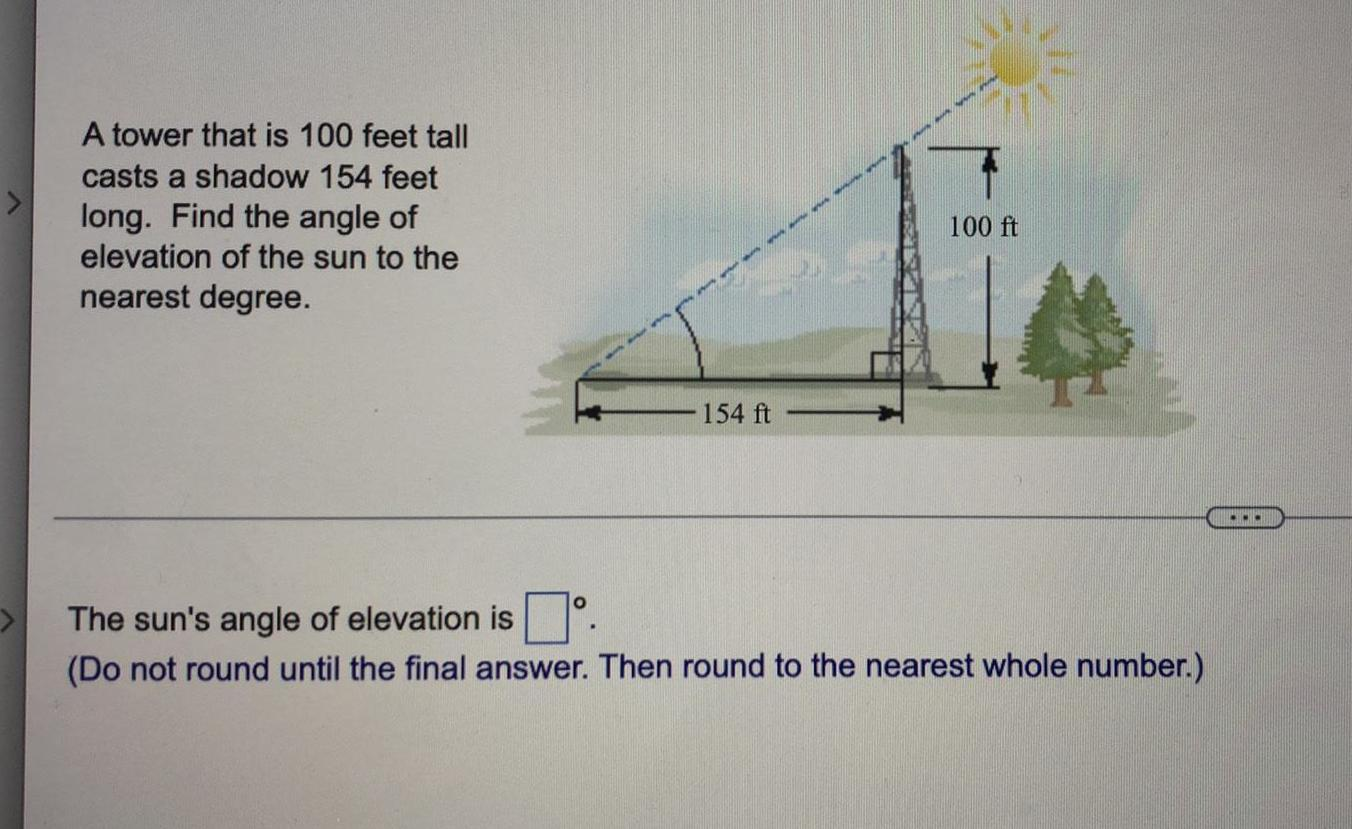Math
Trigonometry
A tower that is 100 feet tall casts a shadow 154 feet long. Find the angle of elevation of the sun to the nearest degree. The sun's angle of elevation is (Do not round until the final answer. Then round to the nearest whole number.)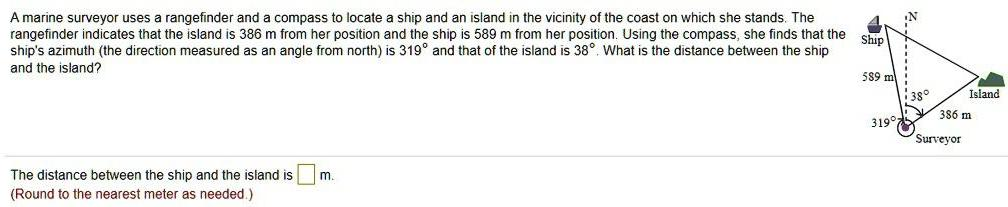Math
Trigonometry
A marine surveyor uses a rangefinder and a compass to locate a ship and an island in the vicinity of the coast on which she stands. The rangefinder indicates that the island is 386 m from her position and the ship is 589 m from her position. Using the compass, she finds that the ship ship's azimuth (the direction measured as an angle from north) is 319° and that of the island is 38°. What is the distance between the ship and the island?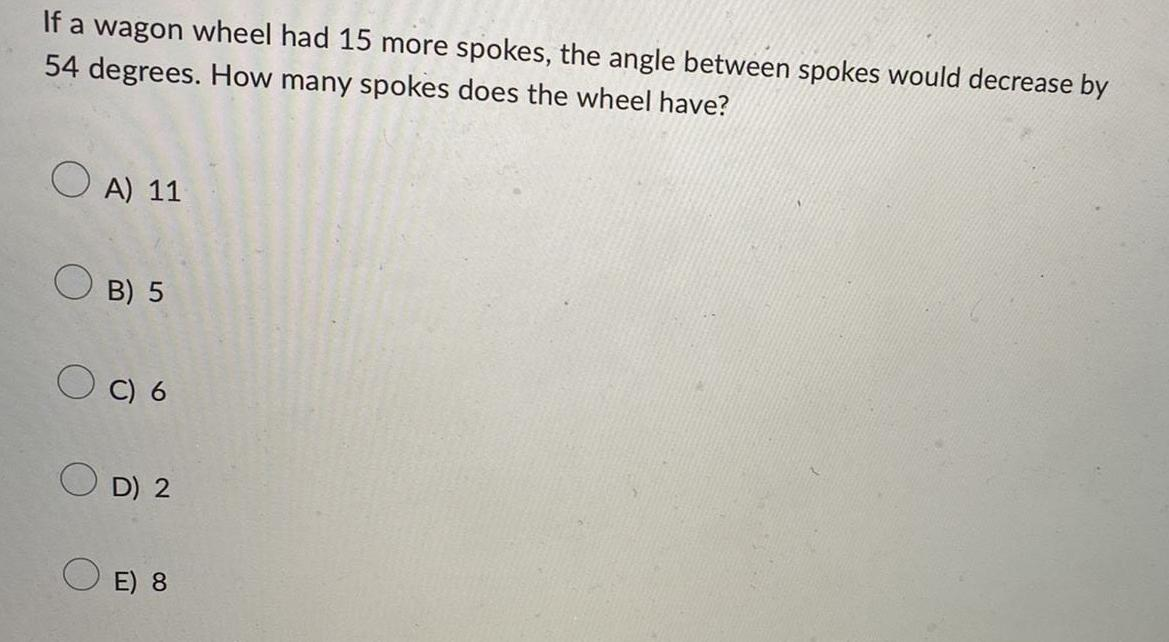Math
Trigonometry
If a wagon wheel had 15 more spokes, the angle between spokes would decrease by 54 degrees. How many spokes does the wheel have? A) 11 B) 5 C) 6 D) 2 E) 8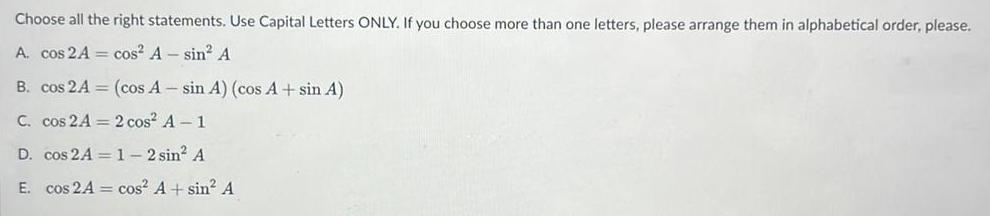Math
Trigonometry
Choose all the right statements. Use Capital Letters ONLY. If you choose more than one letters, please arrange them in alphabetical order, please. A. cos 2A = cos² A- sin² A B. cos 2A = (cos A-sin A) (cos A + sin A) C. cos 2A = 2 cos² A-1 D. cos 2A = 1-2 sin² A E. cos 2A = cos² A+ sin² A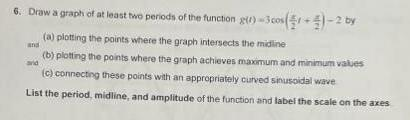Math
Trigonometry
6. Draw a graph of at least two periods of the function gir) -3 cos (x/2t+ x/2)-2 by (a) plotting the points where the graph intersects the midline and (b) plotting the points where the graph achieves maximum and minimum values and (c) connecting these points with an appropriately curved sinusoidal wave List the period, midline, and amplitude of the function and label the scale on the axes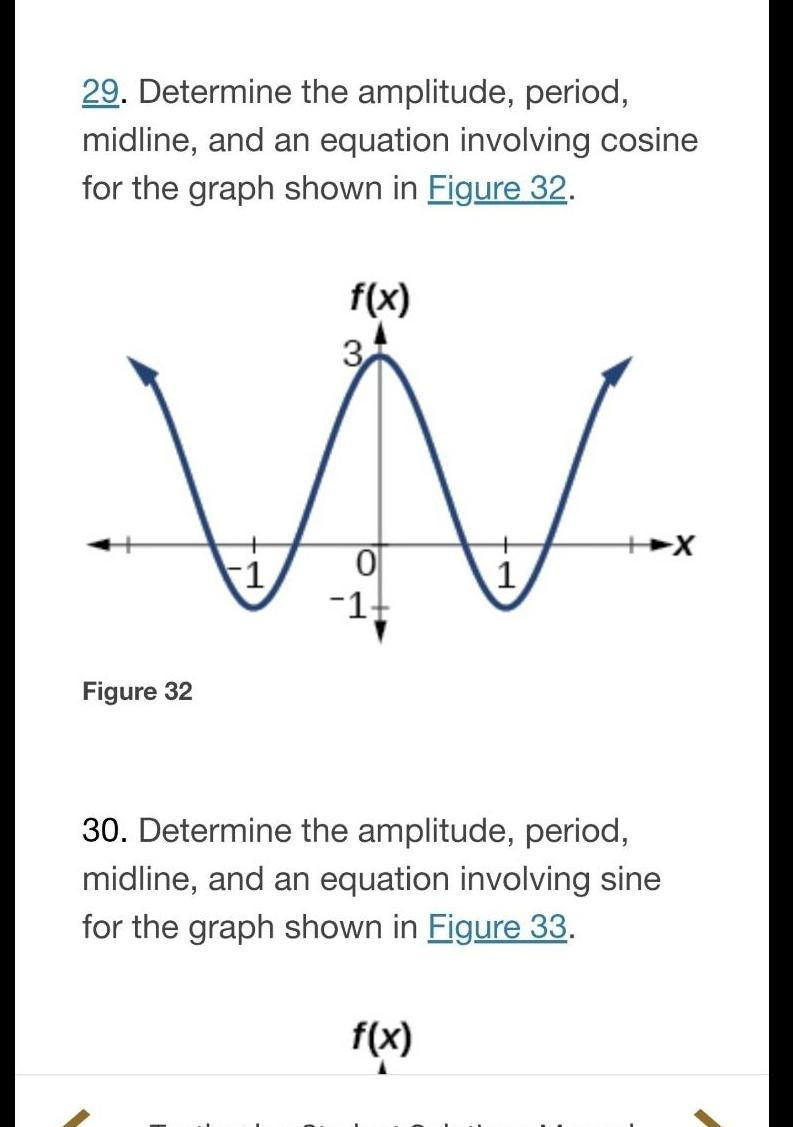Math
Trigonometry
29. Determine the amplitude, period, midline, and an equation involving cosine for the graph shown in Figure 32. 30. Determine the amplitude, period, midline, and an equation involving sine for the graph shown in Figure 33.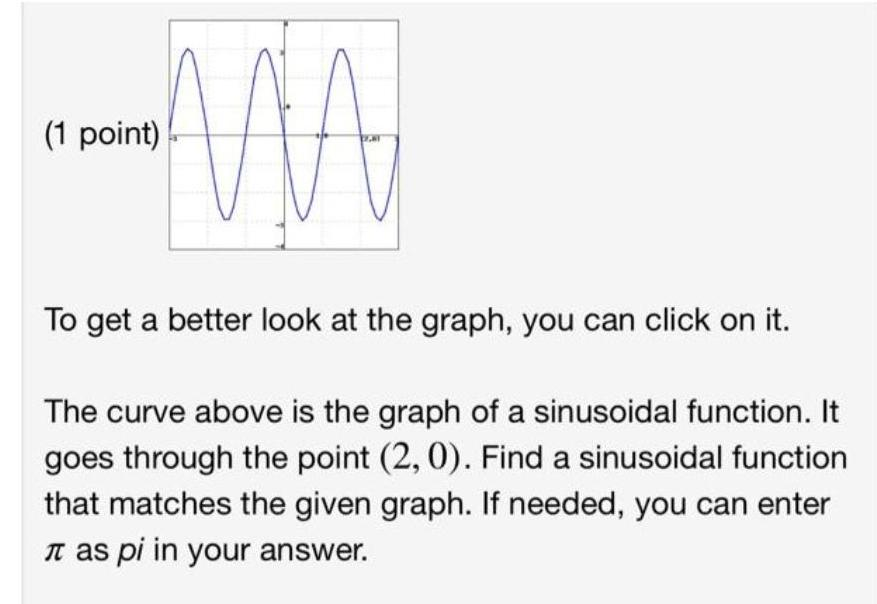Math
Trigonometry
To get a better look at the graph, you can click on it. The curve above is the graph of a sinusoidal function. It goes through the point (2, 0). Find a sinusoidal function that matches the given graph. If needed, you can enter π as pi in your answer.